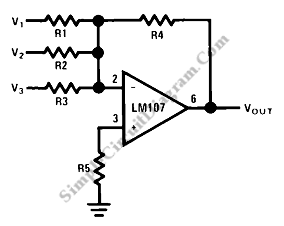# Op-Amp Circuit: Summing Amplifier

This is a summing amplifier circuit. This is an inverting circuit that provides an inverted output equal to the sum of all three input. This circuit has some advantages such as there is no interaction between the operation and inputs, and also it is very easily to implement the weighted averaging and summing on this circuit. Here is the circuit:R1, R2 and R3 are connected in parallel connection. This circuit has gain equal to the ratio of the appropriate input resistor to the feedback resistor. In other words Vout=-R4*(V1/R1 + V2/R2 + V3/R3). If we use the uncompensated amplifier, we must calculate the compensation of this circuit. [Source: National Semiconductor Application Note]